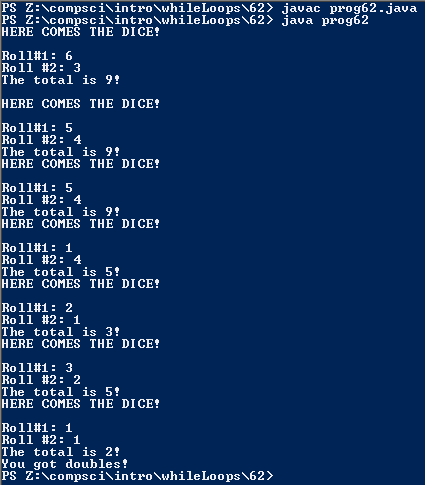# Assignemnt #62, Dice Doubles

## Code

```      /// Name: Alex Pinder
/// Period: 5
/// Program Name: Dice Doubles
/// File Name: prog62.java
/// Date Complete: 1/8/16

import java.util.Scanner;
import java.util.Random;

public class prog62
{
public static void main( String[] args )
{
Random r = new Random();

Scanner keyboard = new Scanner(System.in);

int first, second, together;

first = 1 + r.nextInt(6);
second = 1 + r.nextInt(6);
together = first + second;

System.out.println( "HERE COMES THE DICE!\n\nRoll#1: " + first + "\nRoll #2: " + second + "\nThe total is " + together + "!\n" );

while ( first != second )
{
first = 1 + r.nextInt(6);
second = 1 + r.nextInt(6);
together = first + second;

System.out.println( "HERE COMES THE DICE!\n\nRoll#1: " + first + "\nRoll #2: " + second + "\nThe total is " + together + "!" );
}

System.out.println( "You got doubles!" );
}
}
```

### Picture of the output